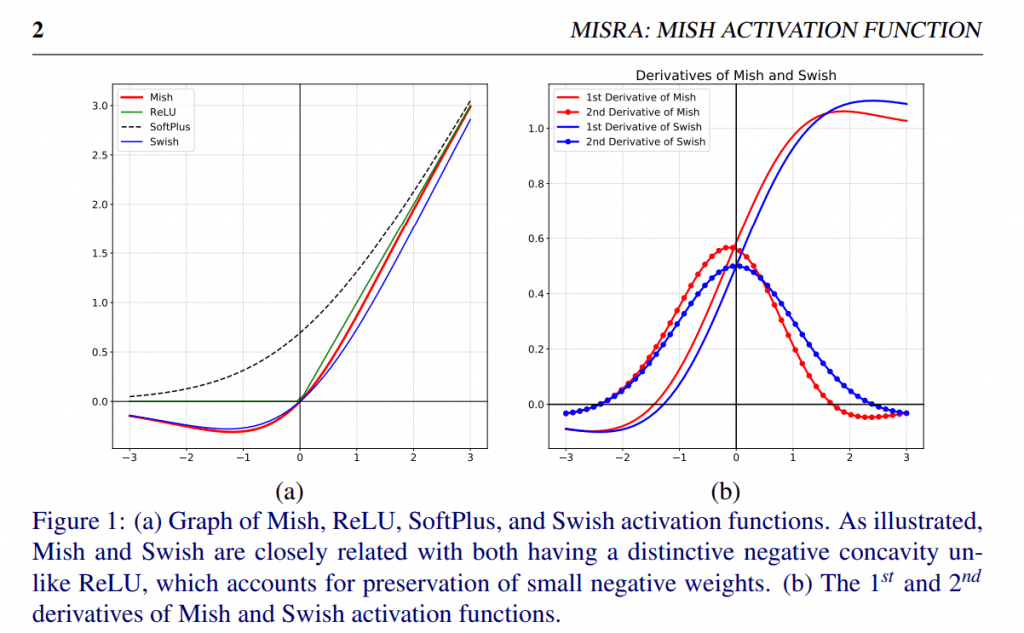# AI Techs :: Learn About Self Regularized Non-Monotonic (Mish) Activation FunctionWhat is Self Regularized Non-Monotonic Activation Function in Neural Networks? How we can use the Mish function in ANN? Where can we use Mish in AI technologies? Let’s remember the activation function and explain these terms.

Activation Function ( phi() ) also called as transfer function, or threshold function that determines the activation value ( a = phi(sum) ) from a given value (sum) from the Net Input Function . Net Input Function, here the sum is a sum of signals in their weights, and activation function is a new value of this sum with a given function or conditions. In another term, the activation function is a way to transfer the sum of all weighted signals to a new activation value of that signal. There are different activation functions, mostly Linear (Identity), bipolar and logistic (sigmoid) functions are used. The activation function and its types are explained well here.

In C++ (in general in most Programming Languages) you can create your activation function. Note that sum is the result of Net Input Function which calculates the sum of all weighted signals. Here activation value of an artificial neuron (output value) can be written by the activation function as below,

By using this sum Net Input Function Value and phi() activation functions, we can code this phi() function. Let’s see some of activation functions in C++; Now Let’s see how we can use Mish Function as in this example formula,

## Self Regularized Non-Monotonic (Mish) Activation Function

Self Regularized Non-Monotonic (Mish) Activation Function is inspired from the Swish activation function is a smooth, continuous, self regularized, non-monotonic activation function. This function is published “Mish: A Self Regularized Non-Monotonic Activation Function” by Diganta Misra in 2019.Graphs from the Mish: A Self Regularized Non-Monotonic Activation Functio by Diganta Misra, 2019

According to this study; “Mish uses the Self-Gating property where the non-modulated input is multiplied with the output of a non-linear function of the input. Due to the preservation of a small amount of negative information, Mish eliminated by design the preconditions necessary for the Dying ReLU phenomenon. This property helps in better expressivity and information flow. Being unbounded above, Mish avoids saturation, which generally causes training to slow down due to near-zero gradients drastically. Being bounded below is also advantageous since it results in strong regularization effects. Unlike ReLU, Mish is continuously differentiable, a property that is preferable because it avoids singularities and, therefore, undesired side effects when performing gradient-based optimization.”

We explained softplus() activation function before. Mish Activation Function can be defined by using softplus() as follows,

Hence, Mish Activation Function can be defined mathematically as follows,

Author compared well Mish, ReLU, SoftPlus and Swish activation function outputs and also compared first and second derivatives of Mish and Swish.

Mish function can be coded in C++ as below,

## A Simple ANN example with Self Regularized Non-Monotonic (Mish) Activation Function in C++

We can simply use this mish function in our generic simple ANN example as below,## Sign up to receive awesome C++ content in your inbox, every day.

We don’t spam! Read our privacy policy for more info.

### Yilmaz Yoru

33+ years of coding with more than 30+ programming languages, mostly C++ on Windows, Android, Mac-OS, iOS, Linux and some other operating systems. Dr. Yilmaz Yoru was born in 1974, Eskisehir-Turkey. He graduated from the department of Mechanical Engineering of Eskisehir Osmangazi University in 1997. One year later he started to work in the same university as an assistant. He received his MSc and PhD degrees from the same department of the same university. He has married and he is a father of a son. Some of his interests are Programming, Thermodynamics, Fluid Mechanics and Artificial Intelligence. He also likes the graphical 2D & 3D design and high-end innovations.